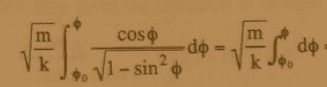# Integrate (cos(p)/(sqrt(1-sin^2p)))dp

## Homework Statement## The Attempt at a Solution

It looks like they think the integration of (cos(p)/(sqrt(1-sin^2p)))dp = 1. I don't see how. I looked at my table of common integrals and I didn't see it. I know that cos^2 + sin^2 = 1 of course but I don't how that helps with the above.

Curious3141
Homework Helper

## Homework Statement## The Attempt at a Solution

It looks like they think the integration of (cos(p)/(sqrt(1-sin^2p)))dp = 1.

No, they're not saying that. They're saying that:

$$\frac{\cos\phi}{\sqrt{1 - \sin^2{\phi}}} = 1$$

(which is true as long as $\phi$ lies in the first or fourth quadrants, a condition which is presumably satisfied by the physical constraints of the problem).

I don't see how. I looked at my table of common integrals and I didn't see it. I know that cos^2 + sin^2 = 1 of course but I don't how that helps with the above.

That identity is the only one you need. Try solving $\cos^2 \phi + \sin^2 \phi = 1$ for $\cos \phi$. Now what happens when you apply that result to the denominator of the integrand?

Last edited:
It is very likely the integral above was preceeded by an integral of the kind
$$\int_{D}\frac{dt}{1-t^2}$$
and a substitution t=sin(x) was performed. I am curious whether you are aware of the term "trigonometric substitution" in an integral? If you do, this should have been no problem. The only identity you need is the Pythagorean identity, which you stated wasn't enough.